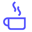a concise shot of dev knowledge
Become a Contributor
Concise shots of dev knowledge

RELATED TAGS

# What is Dynamic Programming?Edpresso Team

Dynamic Programming is a technique to solving complex problems in programming. At its core, the concept is about remembering and making use of answers to smaller “subproblems” that are already solved.

Dynamic Programming (DP) is a powerful technique that makes it possible to solve certain problems considerably more efficiently than a recursive solution would usually be.

This method essentially trades space for time. Instead of calculating all the solution’s different states (taking a lot of time but no space), we take up space for storing solutions of all the sub-problems to save time later. This is called “Memoization.”

Let’s understand this using the classic example of Fibonacci Series:

First few numbers start as: $1, 1, 2, 3, 5, 8, 13...$ and so on.Figure Showing the Function Calls

As we can see in the illustration in order to calculate fib(6), fib(4) is computed 2 times, fib(3) is computed 3 times and fib(2) is computed 5 times. This causes extra function calls for things that have already been calculated, and adds extra time overhead. The problem could simply be solved by storing the results of the subproblems in an array and using the results in place of function calls.

Conveniently, the dynamic programming approach could store the prior results in just 2 variables, because each fib(n) just requires the result of the last two elements.

## Space Complexity: $O(1)$.

Below, a simple solution is shown using recursion and contrasted to one using DP.

## Fibonacci Series Using Recursion

int fib (int n)
{
if (n < 2)
return 1;
return fib(n-1) + fib(n-2);
}

int main()
{
int n = 5;
cout << "Fibonacci of " << n << " = " << fib(n) << "\n";
}

(exponential)

## Fibonacci Series Using DP (Advanced Method)

int fib(int n)
{
int a = 1, b = 1, c;
if (n == 0)
return a;
for (int i = 2; i <= n; i++){
c = a + b;
a = b;
b = c;
}
return b;
}
int main()
{
int n = 5;
cout << "Fibonacci of " << n << " = " << fib(n) << "\n";
}

### Auxiliary Space: $O(1)$

RELATED TAGS

RELATED COURSES

View all Courses

Keep Exploring

Learn in-demand tech skills in half the time